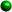Homework Tips for the WeekFactoring Difference of Squares 1. The expression MUST be two terms, each term must be a perfect square, and the expression must be a difference of terms.Here are the perfect squares from 1 to 100: 1, 4, 9, 16, 25, 36, 49, 64, 81, 100 2. We take the square root of the first term and the square root of the last term and the signs in the parentheses must be a plus and a minus. 3. Check your answer by multiplying the binomials using FOIL. For examples and further explanation see: Student Tutorials- Factoring difference of SquaresLook at the video for factoring the difference of two squares. Factoring Trinomials with No Coefficient in the First Term The signs are key. Look at the sign of the third term.If it is positive, the signs in your answer will be the same, either both plus or both minus depending on the middle term.If it is negative, the signs in your answer will be different. If there is NO coefficient in the first term, it is an easier one where by listing the factors of the third term we can see which factors add or subtract to give us the middle term. Always check by FOIL!